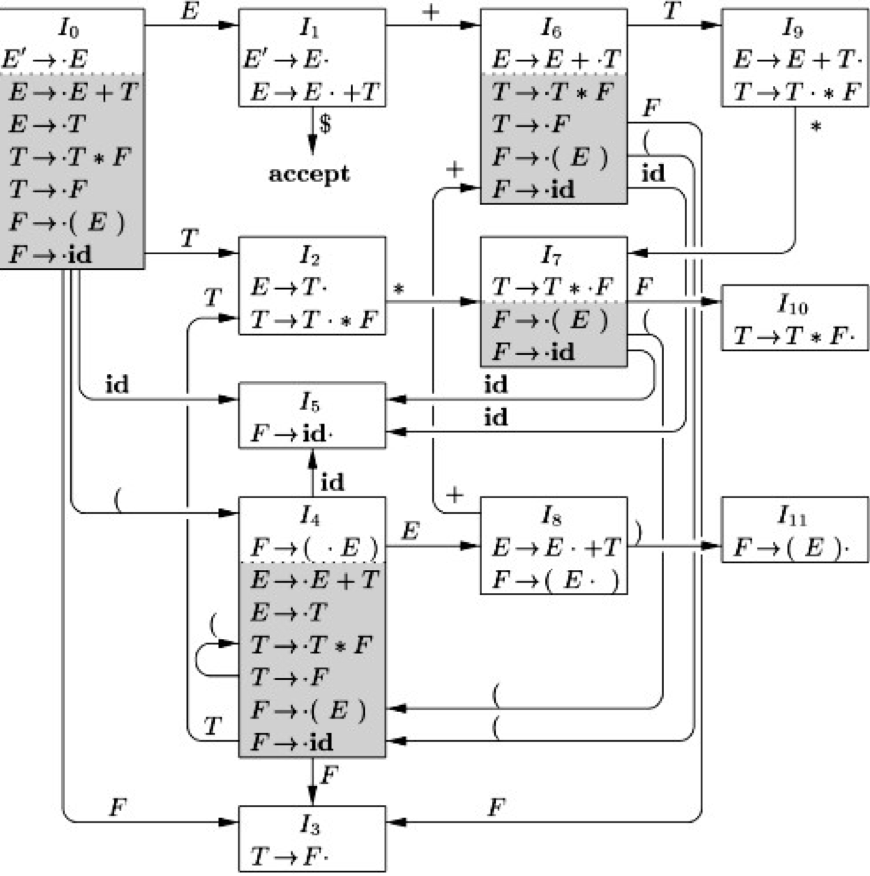$$E \rightarrow E+T|T$$

$$T \rightarrow T*F|F$$

$$F \rightarrow (E) | \textbf{id}$$

$\textbf{id}*\textbf{id}+(\textbf{id}+\textbf{id})*\textbf{id}$移进 $\textbf{id}$没得选
$\textbf{id}$$*\textbf{id}+(\textbf{id}+\textbf{id})*\textbf{id}栈顶归约为 F下一个符号是*，而没有包含 \textbf{id} * 的规则，但栈顶可以归约 F$$*\textbf{id}+(\textbf{id}+\textbf{id})*\textbf{id}$栈顶归约为 $T$下一个符号是$*$，而没有包含$F *$ 的规则，但栈顶可以归约
$T$$*\textbf{id}+(\textbf{id}+\textbf{id})*\textbf{id}移进 *下一个符号是*， 而没有包含 T * 的规则 T*$$\textbf{id}+(\textbf{id}+\textbf{id})*\textbf{id}$移进 $\textbf{id}$栈顶不能归约，只能移进
$T*\textbf{id}$$+(\textbf{id}+\textbf{id})*\textbf{id}栈顶归约为 F下一个符号是+，而没有包含 T *\textbf{id}+ 或者 \textbf{id}+ 的规则，但栈顶可以归约 T*F$$+(\textbf{id}+\textbf{id})*\textbf{id}$栈顶归约为 $T$下一个符号是$+$，而没有包含$T * F+$ 或者 $F+$ 的规则，但栈顶可以归约
$T$$+(\textbf{id}+\textbf{id})*\textbf{id}栈顶归约为 E下一个符号是+，而没有包含T+ 的规则，但栈顶可以归约 E$$+(\textbf{id}+\textbf{id})*\textbf{id}$移进 $+$栈顶不能归约，只能移进
$E+$$(\textbf{id}+\textbf{id})*\textbf{id}移进 (栈顶不能归约，只能移进 E+($$\textbf{id}+\textbf{id})*\textbf{id}$移进 $\textbf{id}$栈顶不能归约，只能移进
$E+(\textbf{id}$$+\textbf{id})*\textbf{id}栈顶归约为 F下一个符号是+，而没有包含 \textbf{id}+ 的规则，但栈顶可以归约 E+(F$$+\textbf{id})*\textbf{id}$栈顶归约为 $T$下一个符号是$+$，而没有包含 $F+$ 的规则，但栈顶可以归约
$E+(T$$+\textbf{id})*\textbf{id}栈顶归约为 E下一个符号是+，而没有 包含 T+ 的规则，但栈顶可以归约 E+(E$$+\textbf{id})*\textbf{id}$移进栈顶不能归约，只能移进
$E+(E+$$\textbf{id})*\textbf{id}移进栈顶不能归约，只能移进 E+(E+\textbf{id}$$)*\textbf{id}$栈顶归约为 $F$下一个符号是$)$，而没有 包含 $\textbf{id})$ 的规则，但栈顶可以归约
$E+(E+F$$)*\textbf{id}栈顶归约为 T下一个符号是)，而没有 包含 F) 的规则，但栈顶可以归约 E+(E+T$$)*\textbf{id}$栈顶归约为 $E$下一个符号是$+$，而没有包含 $T)$ 的规则，但栈顶可以归约
$E+(E+E$$)*\textbf{id}移进栈顶不能归约，只能移进 E+(E+E)$$*\textbf{id}$栈顶归约为 $F$下一个符号是$*$，没有包含 $)*$ 的规则，但栈顶可以归约
$E+F$$*\textbf{id}栈顶归约为 T下一个符号是*，没有包含 F* 的规则，但栈顶可以归约 E+T$$*\textbf{id}$移进这里是tricky的地方，这里考虑到优先级问题必须移进，这样看来**优先移进，不行了再归约可能是处理优先级问题的方案**
$E+T*$$\textbf{id}移进栈顶不能归约，只能移进 E+T*\textbf{id}栈顶归约为 F没有符号了，往死里归约 E+T*F栈顶归约为 T没有符号了，往死里归约 E+T栈顶归约为 E没有符号了，往死里归约 E接受 这是一个漫长的过程，在这个过程中我们发现： • 为了处理运算符的优先级，优先进行移进 • 这和我们编写计算器的方式很像 • 在移进会导致栈中不包含某个可以归约的“前缀”时，必须先归约 • 在栈中的内容不同时，要考虑的是否可以归约的部分也不一样 • 例如在处理栈为 T* 和 E+T* 时，其实都只要考虑 T* 的部分就可以了 那么我们可以看一下有哪些方法解决这些问题。 ## LR(0)项、状态、产生式中的 “\cdot” 与LR(0) 状态机 我们从前面的最后一个问题开始着手。 我们看 E+T* 这里，在这种状态下，我们能用来归约的产生式只有 T \rightarrow T*F 一个，会归约到栈顶上多了一个 T 的状态。1 而在 T* 这里，在这种状态下，我们也能用来归约的产生式只有 T \rightarrow T*F 一个，也只会归约到栈顶上多了一个 T 的状态。 可以看出从“往栈上放一个T的角度来看”这两个状态其实是同一个状态。 ### LR(0)项 我们引入\cdot标记来分割生成式匹配过了的部分和还需要匹配的部分，所以现在这个状态可以写成：$$ T \rightarrow T*\cdot F $$也就代表在这个状态下，已经匹配到了T*，接下来如果能成功地匹配到一个F，就能顺利地归约为栈顶上的一个 T。 像这种标记过匹配到的状态的产生式，我们称之为“LR(0)项”，简称“项”。 ### 项集闭包 我们考虑上面说到的 T \rightarrow T*\cdot F，以及另外两个项：$$ F \rightarrow \cdot(E)  F \rightarrow \cdot \textbf{id} $$我们可以看到在 T \rightarrow T*\cdot F 项的对应状态下，上面两个项所代表的状态其实就是 T \rightarrow T*\cdot F 在试图匹配 F 时的“子状态”或“等价状态”。 那么我们就把这两个状态和原先的 T \rightarrow T*\cdot F 放在一个集合里面，称之为“ T \rightarrow T*\cdot F 的项集闭包”，其中 T \rightarrow T*\cdot F 称为闭包的核心。 那么项集闭包有啥用呢？我们仍然举上面文法的例子： 比如现在栈中有...T*，我们在 T \rightarrow T*\cdot F 状态，我们看到前面有一个'('，此时我们就可以根据这个'(' 和项集闭包中的F \rightarrow \cdot(E)，转到F \rightarrow (\cdot E) 状态，然后去匹配出一个 F，放到栈顶，然后进行 T 的归约。 把所有的项、对应的项集闭包以及状态之间的转换关系列出来，我们就可以建立起一个 LR(0) 状态机：可以看到每一个产生式对应的所有项都会出现在这个状态机里面，而每条边上则指示了在栈顶又被压入某个符号时的状态转换。 其中E'是为了标记我们最终匹配到什么而设置的，其实这个例子来讲说到底就是E。 #### 上述过程的算法描述 这部分看看就行了，看懂了上面的你可以自己想到这些的。 根据龙书，上面的过程的算法描述为： • 求每个LR(0)项，直接往所有每个产生式里能插的地方（包括开头和结尾）插入“\cdot”即可 • 对开始符号所在的项 S'\rightarrow \cdot S，求其闭包 • 寻找每个我们拿到的闭包在某个输入下转换到的项集（称为GOTO函数） • 求法 “If A → α·Bβ is in CLOSURE(I) and B → γ is a production, then add the item B → ·γ to CLOSURE(I), if it is not already there. Apply this rule until no more new items can be added to CLOSURE(I).” • 重复上一步，直到不再有新的项集出现为止 ### 使用 LR(0) 状态机进行匹配 为了存储一路匹配上来时经过的状态，我们需要在分析表中增设一个状态栈。 我不想再画上面那一百万行的巨大表格了，所以我们这次直接用龙书上的例子： 分析\textbf{id} * \textbf{id}： 状态栈符号栈剩余输入动作 I_0$$\textbf{id} * \textbf{id}$根据状态机的标识，转到$I_5$（即压入状态栈），同时移进 $\textbf{id}$
$I_0I_5$$\textbf{id}$$* \textbf{id}$根据状态机的标识，无处可转，进行归约，弹状态栈
$I_0$$F$$* \textbf{id}$弹栈后进行下一步状态转移，转到$I_3$
$I_0I_3$$F$$* \textbf{id}$根据状态机的标识，无处可转，进行归约，弹状态栈
$I_0$$T$$* \textbf{id}$弹栈后进行下一步状态转移，转到$I_2$
$I_0I_2$$T$$* \textbf{id}$根据状态机的标识，转到$I_7$，同时移进 $*$
$I_0I_2I_7$$T*$$\textbf{id}$根据状态机的标识，转到$I_5$，同时移进 $\textbf{id}$
$I_0I_2I_7I_5$$T*\textbf{id}根据状态机的标识，无处可转，进行归约，弹状态栈 I_0I_2I_7$$T*F$弹栈后进行下一步状态转移，转到$I_{10}$
$I_0I_2I_{10}$$T根据状态机的标识，无处可转，进行归约，弹状态栈 I_0I_2$$E$根据状态机的标识，无处可转，进行归约，弹状态栈
$I_0$$E弹栈后进行下一步状态转移，转到I_1 I_0I_1$$E'$接受

### 冲突

• 一个项认为已经可以归约了，另一个项认为还需要移进 $$A \rightarrow a\cdot b$$ $$B \rightarrow c \cdot$$

这称为移进-归约冲突。

• 两个项认为可以归约出不同的东西 $$A \rightarrow b \cdot$$ $$C \rightarrow d \cdot$$

这称为归约-归约冲突。

1